## This Week's Finds in Mathematical Physics (Week 288)

#### John Baez

Happy New Decade! I hope you're doing well. This week I'll say more about rational homotopy theory, and why the difference between equality and isomorphism is important for understanding the weather in space. But first: electrical circuits!

But even before that... guess what this is a picture of: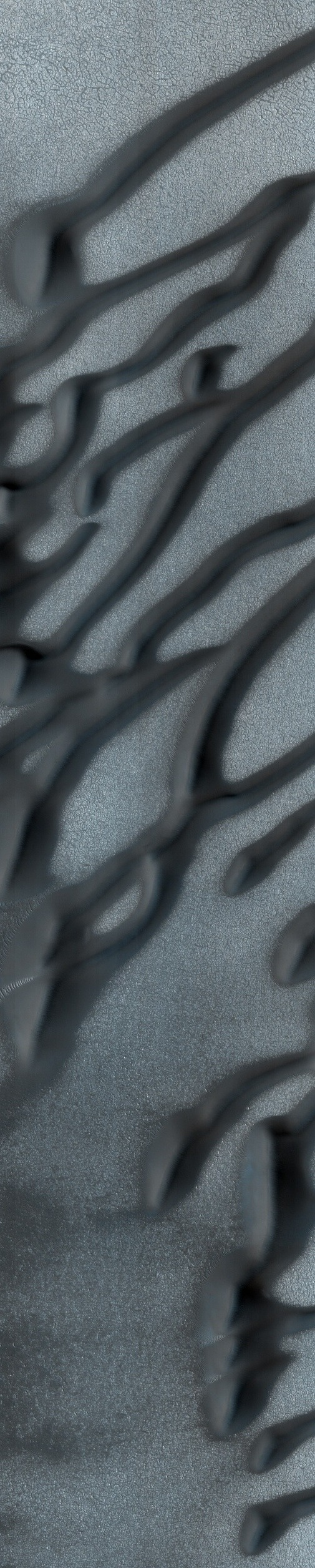Now, what about electrical circuits?

I've been thinking This Week's Finds has become a bit too far removed from its roots in physics. This problem started when I quit working on quantum gravity and started focusing on n-categories. Overall it's been a big boost to my sanity. But I don't want This Week's Finds to be comprehensible only to an elite coterie of effete mathematicians - the sort who eat simplicial presheaves for breakfast and burp up monoidal bicategories.

So, in an effort to prevent This Week's Finds from drifting off into the stratosphere of abstraction, I've decided to talk a bit about electrical circuits. Admittedly, these are less glamorous than theories of quantum gravity. But: they actually work! And there is a lot of nice math involved.

I rarely dare predict what future Week's Finds will discuss, because I know from bitter experience that I change my mind. But lately I've started writing a new way: long stories with lots of episodes, which I can dole out a bit at a time. So I know that for at least a few Weeks I'll talk about electrical circuits - and various related things.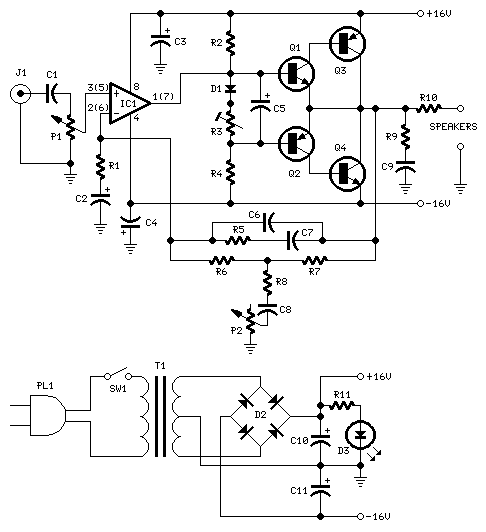10 watt amplifier with bass boost

I've been trying to understand electrical circuits using category theory for a long time. Indeed, Peter Selinger and I are very slowly writing a paper on this subject. The basic inspiration is that electrical circuit diagrams look sort of like Feynman diagrams, flow charts, and various other diagrams that have "inputs" and "outputs". I love diagrams like this! All the kinds I've met so far can be nicely formalized using category theory. For an explanation, try this:

1) John Baez and Mike Stay, Physics, topology, logic and computation: a Rosetta Stone, to appear in New Structures in Physics, ed. Bob Coecke. Available at arXiv:0903.0340.

And after I spent a while thinking about electrical circuits using category theory, I realized that this perspective might shed light on analogies between circuits and other systems.

For example: mechanical systems made from masses and springs!

Indeed, whenever I teach linear differential equations, I like to explain the basic equation describing a "damped harmonic oscillator": for example, a rock hanging on a spring.Then I explain how the same equation describes the simplest circuit made of a resistor, an inductor, and a capacitor - the so-called "RLC circuit". It's a nice easy example of how the same math applies to superficially different but secretly isomorphic problems!

Let me explain. I hope this is a chance to help mathematicians review their physics and ask questions about it over on the n-Category Café.

Let the height of a rock hanging on a spring be q(t) at time t, where q(t) is negative when the end of the spring is down below its equilibrium position. Then making all sort of simplifying assumptions and approximations, we have:

m q"(t) = - c q'(t) - k q(t) + F(t)

where:

• m is the mass of the rock.

• c is the damping coefficient, which describes the force due to friction: we're assuming this force is proportional to the rock's velocity, but points the other way.

• k is the spring constant, which describes the force due to the spring: we're assuming this force is proportional to how much the spring is stretched from its equilibrium position, but points the other way.

• F(t) is the externally applied force, e.g. if you push on the rock.

This equation is just Newton's second law, force equals mass times acceleration. The left side of the equation is mass times acceleration; the right side is the total force.

Now for the analogy. Everything here is analogous to something in an RLC circuit! An RLC circuit has current flowing around a loop of wire with 4 gizmos on it: a resistor, an inductor, a capacitor, and a voltage source - for example, a battery.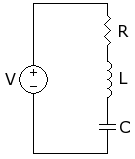I won't say much about these gizmos. I just want to outline the analogy. The amount of current is analogous to the velocity of the rock, so let's call it q'(t). The resistor acts to slow the current down, just as friction acts to slow down the rock. The inductor is analogous to the mass of the rock. The capacitor is analogous to the spring - but according to the usual conventions, a capacitor with a big "capacitance" acts like a weak spring. Finally, the voltage source is analogous to the external force.

So, here's the equation that governs the RLC circuit:

L q"(t) = - R q'(t) - (1/C) q(t) + V(t)

where

• L is the inductance of the inductor.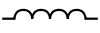inductor

• R is the resistance of the resistor.resistor

• C is the capacitance of the capacitor.capacitor

• V is the voltage of the voltage source.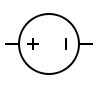voltage source

As you can see, the equation governing the RLC circuit is the same as the one that governs a rock on a spring! True, 1/C plays the role of k, since a capacitor with a big capacitance acts like a spring with a small spring constant. But this is just a difference in conventions. The systems are isomorphic!

We could have fun solving the above equation and pondering what the solutions mean, but that would be the class I teach. Instead, I want to explain how this famous analogy between mechanics and electronics is just one of many analogies.

When I started thinking seriously about electrical circuits and category theory, I mentioned them my student Mike Stay, and he reminded me of the "hydraulic analogy" where you think of an electrical current flowing like water through pipes. There's a decent introduction to this here:

2) Wikipedia, Hydraulic analogy, http://en.wikipedia.org/wiki/Hydraulic_analogy

Apparently this analogy goes back to the early days when people were struggling to understand electricity, before electrons had been observed. The famous electrical engineer Oliver Heaviside pooh-poohed this analogy, calling it the "drain-pipe theory". I think he was making fun of William Henry Preece. Preece was another electrical engineer, who liked the hydraulic analogy and disliked Heaviside's fancy math. In his inaugural speech as president of the Institution of Electrical Engineers in 1893, Preece proclaimed:

True theory does not require the abstruse language of mathematics to make it clear and to render it acceptable. All that is solid and substantial in science and usefully applied in practice, have been made clear by relegating mathematic symbols to their proper store place - the study.

According to the judgement of history, Heaviside made more progress in understanding electromagnetism than Preece. But there's still a nice analogy between electronics and hydraulics.

In this analogy, a pipe is like a wire. Water is like electrical charge. The flow of water plays the role of "current". Water pressure plays the role of "voltage".

A resistor is like a narrowed pipe: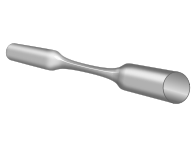An inductor is like a heavy turbine placed inside a pipe: this makes the water tend to keep flowing at the same rate it's already flowing! In other words, it provides a kind of "inertia", analogous to the mass of our rock. Finally, a capacitor is like a tank with pipes coming in from both ends, and a rubber sheet dividing it in two lengthwise: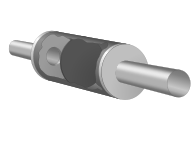When studying electrical circuits as a kid, I was shocked when I first learned that capacitors don't let the electrons through. Similarly, this gizmo doesn't let the water through.

Okay... by now you're probably wanting to have the analogies laid out more precisely. So that's what I'll do. But I'll throw in one more! I've been talking about the mechanics of a rock on a spring, where the motion of the rock up and down is called translation. But we can also study rotation in mechanics. And then we get these analogies:

```
displacement    flow          momentum      effort
q           q'              p            p'

Mechanics       position       velocity       momentum      force
(translation)

Mechanics       angle          angular        angular       torque
(rotation)                     velocity       momentum

Electronics     charge         current        flux          voltage
linkage

Hydraulics      volume         flow           pressure      pressure
momentum
```
The top row lists 4 concepts from the theory of general systems, and my favorite symbols for them, where the prime stands for time derivative - if I could, I'd use a dot. The other rows list what these concepts are called in the subjects listed. So, "displacement" is the general concept which people call "position" in the mechanics of translation. Similarly, "flow" and "effort" correspond to "velocity" and "force", while "momentum" is just "momentum".

I found this chart here:

3) Dean C. Karnopp, Donald L. Margolis and Ronald C. Rosenberg, System Dynamics: a Unified Approach, Wiley, New York, 1990.

I discovered this wonderful book after an intensive search for stuff that makes the analogies between mechanics, electronics and hydraulics explicit. It turns out there's a whole theory devoted to precisely this! It's sometimes called "systems theory" or "network theory". Engineers use this theory to design and analyze systems made out of mechanical, electronic, and/or hydraulic components: springs, gears, levers, pulleys, pumps, pipes, motors, resistors, capacitors, inductors, transformers, amplifiers, and more!

Engineers often describe such systems using a notation called "bond graphs". Bond graphs are reminscent of Feynman diagrams... so they're simply begging to be understood as a branch of applied category theory. In fact, there's an interesting blend of category theory, symplectic geometry and complex analysis at work here. So in the Weeks to come, I'd like to tell you more about bond graphs and analogies between different kinds of systems.

(I'll warn you right now that Karnopp, like most experts on systems theory, use the symbols "f" and "e" for flow and effort, instead of q' and p'. It's more or less impossible to find a unified notation for general systems that doesn't conflict with some existing notation used in the study of some particular kind of system. But since I want to get into symplectic geometry, I want to use some notation that reminds me of that - and for physicists, symplectic geometry is the study of "conjugate variables" like position q and momentum p.)

Okay... enough of this for now.

Last week I introduced the differential graded commutative algebra approach to rational homotopy theory. Next week I'll get into the differential graded Lie algebra approach, filling in another corner of the triangle here:

```                      RATIONAL SPACES
/      \
/        \
/          \
/            \
/              \
DIFFERENTIAL GRADED ------- DIFFERENTIAL GRADED
COMMUTATIVE ALGEBRAS           LIE ALGEBRAS
```
But I realized there's some important stuff I can tell you before we get to that!

Last time I told you how Sullivan defined "rational differential forms" for any topological space X:

First he converted this space into a simplicial set Sing(X).

Then he defined an algebra A of functions that are polynomials with rational coefficients on each simplex of Sing(X).

Then he defined his algebra Ω(A) of rational differential forms, using a general recipe that takes a commutative algebra A and spits out a differential graded commutative algebra.

But towards the end, I admitted that homotopy theorists feel perfectly fine about studying simplicial sets rather than topological spaces. The reason is that both the category of simplicial sets and the category of topological spaces are "model categories" - contexts where you can do homotopy theory. Moreover, these model categories are "Quillen equivalent" - the same in every way that matters for homotopy theory! Don't worry too much if you don't know about model categories and Quillen equivalence. The point is that we have a functor that converts spaces into simplicial sets:

Sing: [topological spaces] → [simplicial sets]

and its right adjoint going back the other way, called "geometric realization":

|   | : [simplicial sets] → [topological spaces]

which we also saw last time. And, these let us freely switch viewpoints between topological spaces and simplicial sets.

So, while in "week286" I defined rational spaces to be specially nice topological spaces, I could equally well have defined them to be specially nice simplicial sets. Taking this viewpoint, we can forget about topological spaces, and think of Sullivan's innovation as a recipe for defining rational differential forms on a simplicial set.

This is a good idea. Among other things, it helps us see more simply what was so new about rational differential forms when Sullivan first discovered them.

What's new is that they give a functor that takes any simplicial set S and gives a differential graded algebra that's commutative and whose cohomology is the rational cohomology of S.

(By which I mean: the rational cohomology of the space |S|.)

You see, it's not so hard to achieve this if we drop our insistence that our differential graded algebra be commutative. This has been known for a long time. You start with your simplicial set S and define a "rational n-cochain" on it to be a function that eats n-simplices and spits out rational numbers. This gives a cochain complex

```       d          d          d
C0(S) ---> C1(S) ---> C2(S) ---> ...
```
where Cn(S) is the vector space of rational n-cochains. This cochain complex is usually called C*(S), where the star stands for the variable n. And there's a standard way to make C*(S) into a differential graded algebra, using a product called the "cup product". But, it's not a differential graded commutative algebra.

Instead, it's only graded commutative "up to chain homotopy". So, we don't have

v ∪ w = (-1)pq w ∪ v

when v is in Cp(S) and w is in Cq(S). But, we do have

v ∪ w = (-1)pq w ∪ v + da(v,w)

where a(v,w) is something that depends on v and w. This is good enough to imply that when we take the cohomology of our cochain complex, we get a graded commutative algebra. This algebra is called H*(S), and the product in here is also called the cup product. You can read a lot about it in basic books on algebraic topology. Here's one that's free online:

4) Allen Hatcher, Algebraic Topology, Section 3.2: Cup Product, available at http://www.math.cornell.edu/~hatcher/AT/ATch3.pdf

The memorably numbered Theorem 3.14 says the cup product is graded commutative.

So you might say: "So, who cares if the cup product of cochains is graded commutative merely up to chain homotopy? When we go to cohomology, that distinction washes away!"

Well, it turns out there can be lots interesting information in this chain homotopy a(v,w). At least, this is true when we do cohomology using the integers or the integers modulo some prime as our coefficients - instead of rational numbers, as we've been doing.

In fact this chain homotopy is the tip of an enormous iceberg! For starters, it satisfies an interesting equation, but only up chain homotopy... and that chain homotopy satisfies an equation of its own, but only up to homotopy, and so on. So, we get a differential graded algebra that's graded commutative up to an infinite series of chain homotopies. Folks call this sort of gadget an "E-algebra".

And when we work over the integers mod some prime, we can squeeze interesting information out of all these chain homotopies. They're called "Steenrod operations". You can use them to distinguish spaces that would be indistinguishable if you merely used their cohomology as a graded commutative algebra!

At least, that's what they say. I don't personally run around using Steenrod operations to distinguish weird spaces that shady characters pull out of their coat pockets on dark streetcorners. Some topologists actually do. But what fascinates me is the subtle distinction between equations that hold "on the nose" and equations that hold only up to homotopy, or up to isomorphism. Sometimes you can "strictify" a gadget where the equations hold only up to homotopy, and get them to hold on the nose. But sometimes you can't.

Once I was giving a talk about n-categories and Roger Penrose was in the audience. I said the most basic fact about n-categories was:

≅ ≠ =

He raised his hand and asked:

≅ ≅ = ?

Very good question! And the answer is: sometimes yes, sometimes no. This is where things get interesting!

So, it's a famous puzzle whether you can find some functorial way to turn a simplicial set S into a differential graded commutative algebra A*(S) whose cohomology is the usual cohomology H*(S). This is called the "commutative cochain problem".

I haven't said it precisely enough yet, since there's a cheap and easy way to solve the version I just stated: just A*(S) to be the cohomology H*(S) itself, with d = 0. What a dirty trick!

To rule out such tricks people demand various extra good properties. For example, the usual cochains C*(S) are "extendible": any cochain on a little simplicial set extends to a cochain on a bigger one. In other words, if

S → T

is an inclusion of simplicial sets, then the corresponding map

C*(T) → C*(S)

is onto. This is definitely not true if we replace C* by H*.

This paper gives a bit of history of the commutative cochains problem:

5) Bohumil Cenkl, Cohomology operations from higher products in the de Rham complex, Pacific J. Math. 140 (1989), 21-33. Available at http://projecteuclid.org/euclid.pjm/1102647247

It gives a somewhat different statement of the problem, which alas I don't understand, and it proves that this version has no solution if we work over the integers. But over the rationals it does, if we take A*(S) to be the rational differential forms on our simplicial set S.

Just so you don't think this is pie-in-the-sky stuff, I should emphasize that this problem actually matters in electrical engineering, where we might triangulate spacetime and study discrete analogues of famous differential equations on the resulting simplicial complex! My friends Robert Kotiuga and Eric Forgy have thought about this a lot.

Here's a nice excerpt from the website of a conference at Boston University. I bet Robert Kotiuga wrote this. It mentions "Whitney forms", which are simplex-wise linear differential forms on a simplicial set. These are closely related to Sullivan's simplex-wise polynomial differential forms.

The analysis of electric circuits, using Kirchhoff's Laws, brought topology into electrical engineering over 150 years ago. Hermann Weyl's reformulation of Kirchhoff's laws in terms of homology over 80 years ago is an abstraction which is proving to be essential in the finite element analysis of three-dimensional electromagnetic fields. It enables computers to be programmed to identify an electrical circuit in an electromagnetic field problem - a task once considered the domain of the engineer's intuition. In "control theory" parlance, circuit theory equations are low frequency model reductions of distributed parameter electromagnetic systems, and homology theory yields the key mathematical tools for obtaining robust numerical algorithms. One aspect of the workshop will deal with large scale homology calculations and the realization of cycles representing generators of integral homology groups as embedded manifolds. The underlying homology calculations involve large sparse integer matrices with remarkable structure even when the underlying finite element meshes are "unstructured". One aim of the workshop is to bring together those performing large scale homology calculations in the context of dynamical systems and point cloud data analysis, with those requiring more geometrical applications of homology groups in electromagnetics.

Over two decades ago, boundary value problems arising in the analysis of quasistatic electromagnetic fields were reinterpreted in terms of Hodge theory on manifolds with boundary. This observation is quite natural when Maxwell's equations are viewed in the context of differential forms and the problem of defining potentials is phrased in terms of de Rham cohomology. This observation, along with the variational formulation of Hodge theory on manifolds with boundary, created a revolution in the finite element analysis of electromagnetic fields. When phrased this way, the most difficult theoretical problems were actually solved in the 1950's by Andre Weil and Hassler Whitney who were concerned with problems in algebraic topology. They had an explicit interpolation formula for turning simplicial cochains into piecewise linear differential forms. This formula gives a chain homotopy between the algebraic complexes involved, and an isomorphism of cohomology rings. Although it took 30 years for Whitney forms to impact engineering practice, once the genie was out of the bottle, there was no way to put it back in. In the early 1990s, Whitney form techniques solved the problem of "spurious modes" appearing in electromagnetic cavity resonator calculations and soon after became widely accepted as an essential tool which is only recently being appreciated in the context of nanophotonics.

It is important to re-examine this Whitney form revolution in the context of recent attempts to develop "discrete exterior calculus," "mimetic discretizations," "compatible discretizations" etc. For example, in algebraic topology it is well known that simplicial cochains do not admit a graded-commutative, associative product analogous to the wedge product on differential forms. This classical result, known as "the commutative cochain problem," is surprising and unintuitive in light of the fact that simplicial cochains admit a graded-commutative, associative product on the level of cohomology, analogous to the one induced by the wedge product in the de Rham complex. The bottom line is that these types of classical results are often ignored by newcomers trying to develop a discrete approach to calculus. Obviously, there is still some important technology transfer to be performed between algebraic topology and numerical analysis! Much of the mathematical work was done by Patodi, Dodziuk and Muller in the 1970's, has been exploited by electrical engineers, but has been largely ignored by applied mathematicians. Although the multiplicative structure on differential forms does not seem to be very important in the context of linear boundary value problems, it seems to play an important role in magnetohydrodynamics. Magnetohydrodynamics, in turn is an essential tool in space physics, in particular, in the growing field of space weather.

So you see, everything is related. The difference between equality and isomorphism matters when you're trying to simulate the weather in space! That's the kind of thing that makes math so fun. Here's the conference webpage:

5) Advanced Computational Electromagnetics 2006 (ACE 'O6), Boston University, http://www.bu.edu/eng/ace2006/

You can learn more here:

6) P. W. Gross and P. Robert Kotiuga, Electromagnetic Theory and Computation: A Topological Approach, Cambridge University Press, 2004.

Someday my discussion of electrical circuits may expand to include some algebraic topology. But all I want to explain now is the usual cup product on the cochains C*(S) for a simplicial set S. We'll need this in the Weeks to come!

Actually, it'll be easier if I work with chains instead of cochains. For us a chain complex will be a list of vector spaces and linear maps

```     d       d      d
C0 <--- C1 <--- C2 <--- ...
```
with d2 = 0. We call the whole thing C*, where now the star is a subscript. I'll show you the usual way to get a chain complex C*(S) from a simplicial set, and then show you a way to comultiply chains. Then you can get the cochains by taking duals:

Cn(S) = Cn(S)*

This will give a way to multiply chains.

Here's how it goes. The idea is that we define the comultiplication directly at the level of simplicial sets and then feed it through a couple of functors. There's a functor

F: [simplicial sets] → [simplicial vector spaces]

and a functor

N: [simplicial vector spaces] → [chain complexes]

Composing these will give the chains C*(S) for a simplicial set S.

The first functor

F: [simplicial sets] → [simplicial vector spaces]

creates the free simplicial vector space on a simplicial set. This functor is symmetric monoidal: it carries products of simplicial spaces to tensor products of simplicial vector spaces. The second functor

N: [simplicial vector spaces] → [chain complexes]

is called the "normalized chain complex" or "normalized Moore complex" functor. This functor is an equivalence of categories! It's almost symmetric monoidal, but not quite, and this is where all the subtlety lies.

The category of simplicial sets has finite products. So, every simplicial set has a diagonal map:

Δ: S → S × S

It also a unique map to the simplicial set called 1, which consists of single 0-simplex:

ε: S → 1

These two maps satisfy the usual axioms of a commutative monoid, written out as commutative diagrams, except with the arrows pointing backwards. So, S is a "cocommutative comonoid" in the category of simplicial sets.

Indeed, whenever you have any category with finite products, every object in it becomes a cocommutative comonoid - and in a unique way!

So far, this is a completely bland fact of life. If we feed our simplicial set S through the functor

F: [simplicial sets] → [simplicial vector spaces]

what happens? Well, because this functor is monoidal it sends comonoids to comonoids. And because it's symmetric monoidal, it sends cocommutative comonoids to cocommutative comonoids. And a cocommutative comonoid in simplicial vector spaces is the same as a "simplicial cocommutative coalgebra".

(I love this kind of stuff, but not everyone does: that's why I save it for the very end of each Week's Finds.)

So, we've turned our simplicial set S into a simplicial cocommutative coalgebra F(S). Now feed this gizmo into the next functor:

N: [simplicial vector spaces] → [chain complexes]

By definition, we get the chains on S:

N(F(S)) = C*(S)

And thanks to the wonders of functoriality, these chains are blessed with a comultiplication

C*(Δ): C*(S) → C*(S) ⊗ C*(S)

and counit

C*(S) → Q

where Q is our ground field, the rationals.

And if the functor N were also symmetric monoidal, C*(S) would also be a cocommutative comonoid, but now in the world of chain complexes. In other words, it would be a "differential graded cocommutative coalgebra". Then, taking duals, the cochains C*(S) would be a DGCA!

But I warned you: things aren't quite so simple.

I said the functor N is almost a symmetric monoidal functor. But not quite.

For starters, it's a "lax monoidal functor", which implies among other things that there's a natural transformation

EZ: N(X) ⊗ N(Y) → N(X ⊗ Y)

This is called the Eilenberg-Zilber map. The word "lax" means that this map isn't necessarily an isomorphism. A lax monoidal functor is good enough to send monoids to monoids. That's important - but it's no use to us now, since we've got a comonoid on our hands!

On the other hand, N is also an "oplax monoidal functor", which implies among other things that there's a natural transformation going the other way

AW: N(X ⊗ Y) → N(X) ⊗ N(Y)

This is called the Alexander-Whitney map. The word "oplax" means that this map isn't necessarily an isomorphism - but now it's going the opposite way. An oplax monoidal functor is good enough to send comonoids to comonoids. Yay!

So, our cochains C*(S) do indeed form a comonoid in the world of chain complexes - that is, "differential graded coalgebra".

However, it's not cococommutative!

To dig deeper into this, I'd need to draw lots of pictures or write lots of formulas, and I don't feel in the mood for that. So, I'll just say what I hope you're thinking: the passage from simplicial vector spaces to chain complexes is quite tricky.

For example, it's sort of frustrating that we have these EZ and AW maps going both ways, but they're not inverses! In fact they come very close. Eilenberg-Zilber followed by Alexander-Whitney is the identity on N(X) ⊗ N(Y). Alas, Alexander-Whitney followed by Eilenberg-Zilber is not the identity on N(X ⊗ Y). But, it's chain homotopic to the identity!

You can read more about the "normalized Moore complex" functor here:

7) nLab, Moore complex, available at http://ncatlab.org/nlab/show/Moore+complex

The fact that it's an equivalence of categories is called the "Dold-Kan correspondence". You can read more about this here:

8) nLab, Dold-Kan correspondence, available at http://ncatlab.org/nlab/show/Dold-Kan+correspondence

And I should point out that while I've been working with vector spaces over the rational numbers, everything I've said about the functors F and N generalize to R-modules for an arbitrary commutative ring R. So, we have

F: [simplicial sets] → [simplicial R-modules]

N: [simplicial R-modules] → [chain complexes of R-modules]

with all the good (or frustrating) properties that I just described. The nLab pages focus somewhat on the case where R = Z, where we get

F: [simplicial sets] → [simplicial abelian groups]

N: [simplicial abelian groups] → [chain complexes of abelian groups]

This is indeed the most fundamental case.

Finally, what about that picture at the beginning? If you're smirking just because you can guess what planet it was taken on, wipe that smile off your face! If I showed you a picture of a city and asked you where it is, would you say "Earth"?

These pictures show linear dunes on the north polar region of Mars: latitude 78 degrees north, longitude 209 degrees east.

(Hmm, on Earth there was a big battle between the British and French to say what longitude counts as "0 degrees". How did it work on Mars?)

Anyway, according to Maria Banks:

This observation shows linear dunes in the north polar region of Mars. Linear or longitudinal sand dunes are elongated, sharp crested ridges that are typically separated by a sand-free surrounding surface.

These features form from bi-directional winds and extend parallel to the net wind direction. In this case, the net wind direction appears to be from the west-southwest. Linear sand dunes are found in many different locations on Earth and commonly occur in long parallel chains with regular spacing.

Superimposed on the surface of the linear dunes are smaller secondary dunes or ripples. This is commonly observed on terrestrial dunes of this size as well. Polygons formed by networks of cracks cover the substrate between the linear dunes and may indicate that ice-rich permafrost (permanently frozen ground) is present or has been present geologically recently in this location.

9) HiRISE (High Resolution Imaging Science Experiments), Linear dunes in the north polar region, http://hirise.lpl.arizona.edu/PSP_009739_2580

Addenda: I thank Richard Lozes and Jonathan vos Post for catching typos. Jesse McKeown pointed out a NASA website that addresses my puzzle about how people settled on a definition of longitude on Mars:

10) NASA, The Martian Prime Meridian - longitude zero, http://www.nasaimages.org/luna/servlet/detail/nasaNAS~4~4~16934~120671:The-Martian-Prime-Meridian----Longi

On Earth, the longitude of the Royal Observatory in Greenwich, England is defined as the "prime meridian," or the zero point of longitude. Locations on Earth are measured in degrees east or west from this position. The prime meridian was defined by international agreement in 1884 as the position of the large "transit circle," a telescope in the Observatory's Meridian Building. The transit circle was built by Sir George Biddell Airy, the 7th Astronomer Royal, in 1850. (While visual observations with transits were the basis of navigation until the space age, it is interesting to note that the current definition of the prime meridian is in reference to orbiting satellites and Very Long Baseline Interferometry (VLBI) measurements of distant radio sources such as quasars. This "International Reference Meridian" is now about 100 meters east of the Airy Transit at Greenwich.) For Mars, the prime meridian was first defined by the German astronomers W. Beer and J. H. Mädler in 1830-32. They used a small circular feature, which they designated "a," as a reference point to determine the rotation period of the planet. The Italian astronomer G. V. Schiaparelli, in his 1877 map of Mars, used this feature as the zero point of longitude. It was subsequently named Sinus Meridiani ("Middle Bay") by Camille Flammarion. When Mariner 9 mapped the planet at about 1 kilometer (0.62 mile) resolution in 1972, an extensive "control net" of locations was computed by Merton Davies of the RAND Corporation. Davies designated a 0.5-kilometer-wide crater (0.3 miles wide), subsequently named "Airy-0" (within the large crater Airy in Sinus Meridiani) as the longitude zero point. (Airy, of course, was named to commemorate the builder of the Greenwich transit.) This crater was imaged once by Mariner 9 (the 3rd picture taken on its 533rd orbit, 533B03) and once by the Viking 1 orbiter in 1978 (the 46th image on that spacecraft's 746th orbit, 746A46), and these two images were the basis of the Martian longitude system for the rest of the 20th Century. The Mars Global Surveyor (MGS) Mars Orbiter Camera (MOC) has attempted to take a picture of Airy-0 on every close overflight since the beginning of the MGS mapping mission. It is a measure of the difficulty of hitting such a small target that nine attempts were required, since the spacecraft did not pass directly over Airy-0 until almost the end of the MGS primary mission, on orbit 8280 (January 13, 2001). In the left figure above, the outlines of the Mariner 9, Viking, and Mars Global Surveyor images are shown on a MOC wide angle context image, M23-00924. In the right figure, sections of each of the three images showing the crater Airy-0 are presented. A is a piece of the Mariner 9 image, B is from the Viking image, and C is from the MGS image. Airy-0 is the larger crater toward the top-center in each frame. The MOC observations of Airy-0 not only provide a detailed geological close-up of this historic reference feature, they will be used to improve our knowledge of the locations of all features on Mars, which will in turn enable more precise landings on the Red Planet by future spacecraft and explorers.

For more discussion, visit the friendly and welcoming n-Category Café.

If you haven't found something strange during the day, it hasn't been much of a day. - John Wheeler

© 2010 John Baez
baez@math.removethis.ucr.andthis.edu